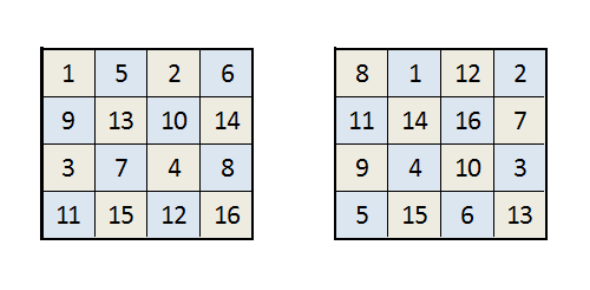# Integers Quiz! Lets See How Good You Are With Numbers

Approved & Edited by ProProfs Editorial Team
The editorial team at ProProfs Quizzes consists of a select group of subject experts, trivia writers, and quiz masters who have authored over 10,000 quizzes taken by more than 100 million users. This team includes our in-house seasoned quiz moderators and subject matter experts. Our editorial experts, spread across the world, are rigorously trained using our comprehensive guidelines to ensure that you receive the highest quality quizzes.
| By Catherine Halcomb
C
Catherine Halcomb
Community Contributor
Quizzes Created: 1516 | Total Attempts: 5,427,589
Questions: 15 | Attempts: 197SettingsAn integer is colloquially defined as a number that can be written without a fractional component. For example, 21, 4, 0, and −2048 are integers, while 9.75, 512, and √2 are not.
Type the correct answer in the box. Make sure to always type the integer in front of the digits, without a space; the computer will be marking the answers.
Example: +5 OR -11 OR -1

• 1.

### (-4) + (6) = (alway put the + or - infront of the digit)

Explanation
When you add a positive number to a negative number, the result will be the sum of their absolute values with the sign of the larger number. In this case, the absolute value of -4 is 4 and the absolute value of 6 is 6. Since 6 is larger, the result will have a positive sign. Therefore, (-4) + (6) equals +2.

Rate this question:

• 2.

### (5) – (-2) =

Explanation
The given expression is a subtraction problem where we subtract a negative number from a positive number. When we subtract a negative number, it is the same as adding the positive value of that number. So, subtracting -2 is equivalent to adding 2. Therefore, the result of the expression is +7.

Rate this question:

• 3.

### (-3) x (-3) =

Explanation
When multiplying two negative numbers, the result is always positive. In this case, multiplying -3 by -3 yields a positive 9.

Rate this question:

• 4.

### -8 ÷ (+2) =

Explanation
When dividing a negative number by a positive number, the result is always negative. In this case, -8 divided by +2 equals -4.

Rate this question:

• 5.

### (-3) x (-4) ÷ (-2) =

Explanation
When multiplying two negative numbers, the result is always positive. In this case, (-3) multiplied by (-4) equals 12. Then, dividing 12 by (-2) gives us -6. Therefore, the correct answer is -6.

Rate this question:

• 6.

### (-4) + (-1) – (+4) =

Explanation
The given expression involves adding two negative numbers and subtracting a positive number. When we add two negative numbers, the result is a negative number. Then, when we subtract a positive number from a negative number, the result is also a negative number. Therefore, the expression (-4) + (-1) - (+4) equals -9.

Rate this question:

• 7.

### (+5) (-5) =

Explanation
The given expression (+5) (-5) can be solved by multiplying the positive number 5 with the negative number 5. When a positive number is multiplied by a negative number, the result is always negative. Therefore, the product of (+5) (-5) is -25.

Rate this question:

• 8.

### (4) – (5) – (-2) =

Explanation
The given expression involves subtracting a positive number (-2) from a negative number (5), which results in a negative number (-7). Subtracting this negative number (-7) from another positive number (4) gives a positive number (+1).

Rate this question:

• 9.

### (-9) ÷ (-3) =

Explanation
When dividing a negative number (-9) by another negative number (-3), the result will be positive. This is because when dividing two numbers with the same sign, the quotient will always be positive. In this case, -9 divided by -3 equals +3.

Rate this question:

• 10.

• 11.

### +3 + (-2)3 x (-2) =

Explanation
The given expression involves addition, multiplication, and exponentiation. According to the order of operations, we first evaluate the exponentiation: (-2)3 = -8. Then, we multiply -8 by -2, which gives us +16. Finally, we add 3 to +16, resulting in +19.

Rate this question:

• 12.

### -2 (-2 + 3 ) + 6 =

Explanation
The given expression can be simplified by following the order of operations (PEMDAS/BODMAS). First, we evaluate the expression inside the parentheses: -2 + 3 = 1. Then, we multiply -2 by 1, resulting in -2. Finally, we add 6 to -2, giving us a final answer of +4.

Rate this question:

• 13.

### (-8) ÷ (2) - (+3) x (-3) =

Explanation
The given expression involves division, multiplication, and addition. According to the order of operations, we first perform the division, which is -8 divided by 2, resulting in -4. Then, we multiply -4 by -3, giving us +12. Finally, we add +12 to +3, resulting in +15. Therefore, the correct answer is +15, not +5.

Rate this question:

• 14.

### (-4) – (5) x (-2) all divided by (-2)

Explanation
The expression (-4) - (5) x (-2) all divided by (-2) can be simplified by following the order of operations (PEMDAS). First, we perform the multiplication: (-5) x (-2) = 10. Then, we perform the subtraction: (-4) - 10 = -14. Finally, we divide the result by (-2): -14 ÷ (-2) = 7. Therefore, the correct answer is 7.

Rate this question:

• 15.

### (-2) + (-2) (-3) (-1) (0) (-2) (-3) =

Explanation
The given expression is a multiplication of negative numbers. When multiplying negative numbers, the result is positive if there is an even number of negative signs, and negative if there is an odd number of negative signs. In this case, there are 6 negative signs, which is an even number. Therefore, the result of the expression is positive, and since the expression only contains -2, the answer is -2.

Rate this question:

Related TopicsBack to top